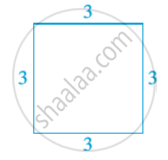Tamil Nadu Board of Secondary EducationSSLC (English Medium) Class 9th

# Polynomials in One Variable

#### notes

A particular type of algebraic expression, called polynomial. The letters x,y,z etc. to denote variables where as 2x , 3x , -x , -1/2x are algebric expressions. . The values of the constants remain the same throughout a particular situation, that is, the values of the constants do not change in a given problem, but the value of a variable can keep changing.
Now, consider a square of side 3 units in following fig.The perimeter of a square is the sum of the lengths of its four sides. Here, each side is 3 units. So, its perimeter is 4 × 3, i.e., 12 units. The perimeter is 4 × 10, i.e., 40 units. In case the length of each side is x units  in following  fig.the perimeter is given by 4x units. So, as the length of the side varies, the perimeter varies.
It is x × x = x^2 square units. x^2 is an algebraic expression. You are also familiar with other algebraic expressions like 2x, x^2 + 2x, x^3 – x^2 + 4x + 7. Note that, all the algebraic expressions we have considered so far have only whole numbers as the exponents of the variable. Expressions of this form are called polynomials in one variable.

In the polynomial x^2 + 2x, the expressions x^2 and 2x are called the terms of the polynomial.There are many terms of the polynomial.Each term of a polynomial has a coefficient. So, in –x^3 + 4x^2 + 7x – 2, the coefficient of x^3 is –1, the coefficient of x^2 is 4, the coefficient of x is 7 and –2 is the coefficient of x^0 (Remember, x^0 = 1).

2 is also a polynomial. In fact, 2, –5, 7, etc. are examples of constant polynomials. The constant polynomial 0 is called the zero polynomial.
If the variable in a polynomial is x, we may denote the polynomial by p(x), or q(x), or r(x), etc. for example : p(x) = 2x^2 + 5x – 3
q(x) = x_3 –1.
A polynomial can have any (finite) number of terms.
Polynomials having only one term are called monomials (‘mono’ means ‘one’). example : 2x , 2.
Polynomials having only two terms are called binomials (‘bi’ means ‘two’) example : p(x) = x + 1,  q(x) = x^2 – x .
Polynomials having only three terms are called trinomials (‘tri’ means ‘three’). example : p(x) = x + x^2 + π,  q(x) = sqrt2 + x – x^2
The highest power of the variable in a polynomial as the degree of the polynomial.
For example 3x^7 the term with the highest power of x in this term is 7.
The degree of a non-zero constant polynomial is zero.

A polynomial of degree two is called a quadratic polynomial.
For example : 4y + 5y^2 ,  5 – y^2
A polynomial of degree three a cubic polynomial.
For example : , 5x^3 + x^2 , 2x^3 + 1.

If you would like to contribute notes or other learning material, please submit them using the button below.

### Shaalaa.com

Polynomials in One Variable [00:03:30]
S
0%